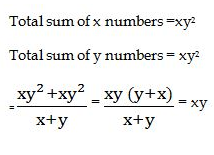# RRB ALP 2018 Practice Test Papers | Arithmetic Questions (Day-54)

Dear Aspirants, Here we have given the Important RRB ALP & Technicians Exam 2018 Practice Test Papers. Candidates those who are preparing for RRB ALP 2018 can practice these Arithmetic Questions to get more confidence to Crack RRB 2018 Examination.

[WpProQuiz 2725]

Click “Start Quiz” to attend these Questions and view Solutions

1. Tap P can fill a tank in 60 mins. Tap Q can fill the same tank in 30 mins. Tap R can empty the tank in 2 hours. If the 3 taps are opened simultaneously how long the tank take to fill?
1. 20 mins
2. 30 mins
3. 24 mins
4. 25mins
1. Find the value of

8888+888+88+8 = ?

1. 9872
2. 9782
3. 9792
4. 9862
1. The least number which when divided by 5, 6, 7 and 8 leaves a remainder 3, but when divided by 9 leaves no remainder is
1. 1677
2. 1683
3. 2523
4. 3363
1. The square root of (2722 – 1282)
1. 256
2. 200
3. 240
4. 144
1. The average of x numbers is y2 and the average of y numbers is x2. So the average of all the numbers taken together is
1. x2 + y2
2. xy
3. x + y
4. x/y
1. A land owner sells his land with the profit of 25%. If the selling price of the land is 1,25,000, then what is the cost price of the land?
1. 1,50,00
2. 1,52,000
3. 1,00,000
4. 1,20,000
1. tan 5°. tan 45°. 4 tan 85° = ?
1. 4/√3
2. 4√3
3. √3
4. 4
1. Measure of each interior angle of a regular polygon can never be
1. 150°
2. 105°
3. 108°
4. 144°
1. Find the value of x

280 + 45% 0f 300 + 5x – 400

1. 15
2. 25
3. 23
4. 20
1. A car goes a speed of 40 kmph and return with the speed of 60 kmph. What is the average speed of the whole journey?
1. 24 kmph
2. 60 kmph
3. 48 kmph
4. 12 kmph

P – 1/60          Q – 1/30                     R- 1/120

P+Q+R = 1/60 + 1/30 – 1/120 =(2+4 – 1)/120 = 5/120 = 24 mins

8888+888+88+8 = 9872

LCM of 5, 6, 7 and 8 = 840

Required number = (840k + 3) which is divisible by 9.

Now, 840k +3 = 93 ×9K +(3k+3)

When k = 2, 3K+3 =9, which is divisible by 9

∴Required number = 840 ×2 +3 = 1683

√2722 – 1282 = √(272 – 128)(272+128)

= √(400×144) =√(20×20×12×12 )= 20×12 = 240(1,25,00× 100)/125 = 10,00,00

tan5°.Tan45°. 4 tan 85° = (tan90 – 5°) .tan 45°. 4 tan 85°

= cot 85°. 4 tan 85° .tan 45°

=4(1). 1 = 4

The measure of each interior angle of a regular polygon is always 180(n — 2)/n degrees, where n represents the number of sides of the polygon. The measure of each exterior angle of a regular polygon is 360/n degrees

280 + 45% 0f 300 + 5x – 300

= 280 + 135 + 5x – 300 = 415 – 300 +5x

5x =115

X = 23

Average speed = (2xy)/x + y

= (2 × 40 ×60) / 100

= 4800/100 = 48

RRB ALP 2018 – “All in One” Study Materials and Practice Sets